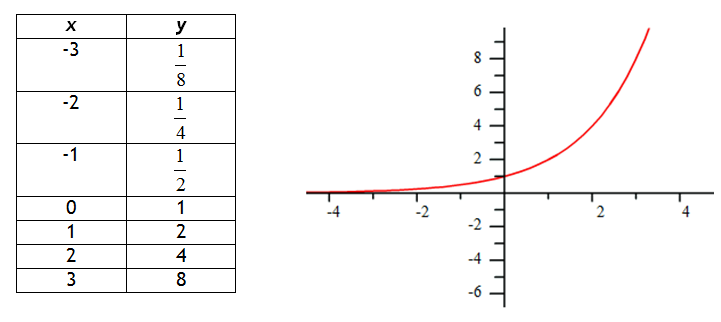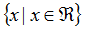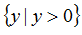Growth

The exponential parent function is y = kx where k is a constant. The easiest way to begin looking at this pattern is to make a table.

Recall from the Families of Functions Unit that the function g(x) = 2x would create the following table and graph:Notice that the graph increases more rapidly as x increases. The left side of the graph is approaching, but never touches the line y = 0, which is the graph's asymptote. Therefore, the domain of this function isand the range of the function is.